# Dataset of numerical model for enhancing stimulated Brillouin scattering in optical fibers

## Cite this dataset

Abdul-Rashid, Hairul; Yeap, Kelvin; Emami, Siamak (2021). Dataset of numerical model for enhancing stimulated Brillouin scattering in optical fibers [Dataset]. Dryad. https://doi.org/10.5061/dryad.kd51c5b4w

## Abstract

Stimulated Brillouin scattering (SBS) is useful, among others for generating slow light, sensing and amplification. SBS was previously viewed as a penalty due to the limitation on optical power in high-powered photonic applications. However, considering the many possible applications using SBS, it is now of interest to enhance SBS in areas of Brillouin frequency shift together with Brillouin Gain. A numerical model, using a fully vectorial approach, by employing the finite element method, was developed to investigate methods for enhancing SBS in optical fiber. This paper describes the method related to the numerical model and discusses the analysis between the interactions of horizontal, shear and hybrid acoustic modes; and optical modes in optical fiber. Two case studies were used to demonstrate this. Based on this numerical model, we report the influence of core radius, clad radius and effective refractive index on the Brillouin frequency shift and gain. We observe the difference of Brillouin shift frequency between a normal silica optical fiber and that of a tapered fiber where nonlinearities are higher. Also observed, the different core radii used and their respective Brillouin shift. For future work, the COMSOL model can also be used for the following areas of research, including simulating “surface Brillouin shift” and also to provide in-sights to the Brillouin shift frequency vB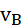of various structures of waveguides, e.g circular, and triangular, and also to examine specialty fibers, e.g. Thulium and Chalcogenide doped fibers, and their effects on Brillouin shift frequency.

## Methods

We present a numerical model to optimize Brillouin frequency shift and gain based on various core diameters of the tapered region of silica microfiber structures. To further understand the interactions, the COMSOL model used is capable of modelling solutions in the given structures of the optical fiber. Previous research was mainly done to enhance performance on spatial resolution and also sensing range, but there have not been many insights for gain. The ability to increase the Brillouin gain coefficient has opened new opportunities to control their interaction, and several new industrial and commercial applications. In this research work, we demonstrate the numerical model for a microfiber design that is expected to increase Brillouin gain coefficient. The fully vectorial method, developed in COMSOL, is used in this case to determine the contributions of various optical fibre parameters towards SBS, thus aiding the design of microfibers with enhanced SBS performance. The equation below is used for the analysis of light guidance where H is the full vectorial magnetic field, * represents the complex conjugate and transpose, ω2 denotes the eigenvalue where ω is the optical angular frequency of the wave and ε and µ are the permittivity and permeability, respectively (21).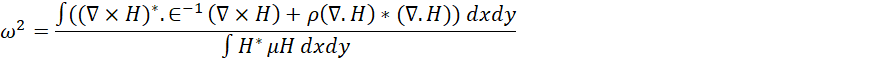(1)

Equation (1) solves for the propagation constant of optical modes in optical waveguides, which can also guide acoustic mode. The propagation constant of the optical wave, β, is defined as βoptic=2πneff /λ. There are two basic types of acoustic waves, namely shear and longitudinal acoustic waves. Shear waves are associated with dominant material dispersion in the transverse directions, which is perpendicular to the direction of propagation, taken here as the z-axis. On the other hand, for a longitudinal wave, expansion and contraction of the wave is associated with particle movements along the z direction which is in parallel to the wave propagation. However, acoustic wave propagating through a waveguide can be a combination of shear and longitudinal acoustic waves. Brillouin frequency shift of the Stokes wave is given as f = 2neff Vac/ λ, where, Vac is the acoustic velocity. The acoustic wave satisfies Hooke’s Law (12) which relates to the stress (tensor) and strain (force) of the waveguiding materials. Electric field associated with a high power optical signal causes molecular movements due to electrostriction process (13). Such a material movement can generate acoustic waves that leads to density variation along the waveguide. The time and space dependent density variation changes the refractive index profile and produces a moving optical grating. This grating can reflect incoming light when its wavelength matches the spatial period of the gratings generated by the acoustic wave. Above a threshold power, if phase matching conditions are satisfied, it can inhibit forward guidance of the incoming light. The backward scattered reflected wave is frequency shifted, which explains the occurrence of the Stokes Wave. The relationship between optic and acoustic propagation for phase matching condition can be given as: Kacoustic =2βoptic where Kacoustic is the acoustic propagation constant and this will be double of the βoptic, the optic propagation constant.

For the SBS characterization in the optical fiber, both its guided optical and acoustic modes can be obtained using the FEM. The n eff for the optical mode in a fibre for a given radius is first calculated using H-field based FEM model. Eigenvector and eigenvalue of acoustic waves are also obtained and then the acoustic mode patterns are generated. At phase matched conditions, the acoustic wave propagation constant is double the value of the optical wave propagation constant: Kacoustic =2β optic.

In this research work, the fully vectorial approach was used to solve the optical wave equations for neff using the commercial COMSOL software. The optical parameters E(x,y) and neff   , are obtained by solving the equation below: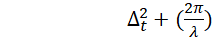2(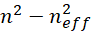)E=0                                                                  (2)

Where ∆t is transverse Laplacian operator in the (x,y) direction, while neff is the effective refractive index of fundamental optical mode (9), directly related to Brillouin frequency shift via the Bragg condition (10). The acoustic wave, which consists of the stress and strain components, are governed by Hooke’s Law (12) whereby solving the equation below would yield its displacement.

Tij  = cijklSkl ; 𝑖,𝑗, 𝑘𝑙 = 𝑥, 𝑦, 𝑧                                                                  (3)

where T denotes the stress field and S represents the force field which is equivalent to partial differentiation of displacement. cijkl is the tensor relation of elastic stiffness where i, j, kl are equivalent to propagation in 𝑥, 𝑦 and 𝑧 direction respectively (22). For an isotropic medium with uniform wave propagation, the elastic stiffness constant is given by the longitudinal and shear velocity that is dependent on the material properties of the optical fiber core and cladding (12).

For the purpose of calculating the Brillouin gain, the overlap factor from the fully vectorial approach was used. The overlap between optical wave and acoustic wave is given in equation (4) below (21).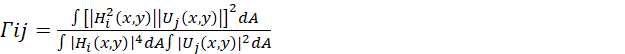(4)

where Hi (x, y) is the fundamental mode in optical wave and Uj (x, y) is the displacement vector of acoustic wave. Optic-acoustic wave overlap factor is influenced by the acoustic wave’s strain field and refractive index of the optical fiber (11). Both the optical and acoustic wave vectors have to be normalized to calculate the overlap factor.

The Brillouin gain coefficient is represented by the equation below (23):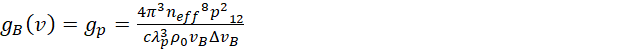(5)

Where ρ0 is fiber core density, p12 is elasto-optic coefficient which contributes to the periodic light scattering and is FWHM of acoustic wave in SBS (23).

COMSOL PARAMETERS

Table 1: Tapered fiber parameters

 Case Study Core (Diameter) Cladding (diameter) Refractive Index (Core/Clad) Density of material ρ (kg/m3) Wavelength 1 2µm 6µm 1.4502/1.445 2202 1550nm 2 4µm 12µm

Table 2: Acoustic Velocity Parameters for Acoustic Model Simulation (Sriratanavaree et al., 2014) (21)

 Mode Region Vl (m/s) Vs (m/s) Shear acoustic Core 5933 3625 Shear acoustic Clad 5933 3764 Longitudinal acoustic Core 5736 3764 Longitudinal acoustic Clad 5933 3764 Hybrid acoustic Core 5933 3625 Hybrid acoustic Clad 5933 3764

METHOD IN COMSOL

Based on the parameters in table 1, SBS characterization using the fully vectorial approach was performed on various core diameters of the tapered region of the silica microfiber and was verified against earlier results by H.J Lee (23). The neff were calculated for all core diameters. Refractive index for the core and clad used were 1.4502 and 1.445 respectively considering the measured values in a typical single mode fiber (21). Following that, three cases of acoustic wave, shear, longitudinal and hybrid behavior were analyzed.

Optical Mode Solver

To obtain the neff results for the 2 case studies covered in this research, the optical mode equation was solved using the COMSOL RF module. In the RF module the electromagnetic waves, frequency domain physics engine was used. The geometric structures of the fibers were generated using the 2 dimensional space dimension. From there, the parameters as denoted in table 1 were entered into the solver. The density, refractive index of both the core and clad values are declared under the materials section. Thereafter, the mode analysis frequency is set to the desired wavelength of 1550nm and the perfect electrical conductor boundary condition was used. As with all FEM solvers meshing is required. For this case, the triangular mesh and the “finer” element size was used. The effective mode index or neff for the fundamental mode can thereafter be obtained after computing the solver.

Acoustic Mode Solver

As for the acoustic model, the acoustic waves of shear and longitudinal were evaluated independently to record the findings. Thereafter, the hybrid acoustic wave across the fiber in which both shear and longitudinal acoustic waves co-exist were examined. The hybrid mode model was the model used to determine the Brillouin Shift Frequency vB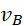as both these waves propagate together in real life conditions. Table 2 shows the velocity assigned for core clad region in each respective case. In the case of pure shear acoustic, longitudinal velocity for the core region were made equivalent to 5736 m/s, this is to prevent interruption of longitudinal acoustic wave. Similarly, for pure longitudinal acoustic, the shear acoustic of the core was made to 3625 m/s. For the hybrid acoustic wave, the velocities of both the longitudinal and shear waves of the clad are defined to be slightly higher than the core (21). This is to prevent interruption of acoustic wave between the two regions. The core-clad ratio was taken to be 1:3 so that the clad region is long enough to prevent wave reflection from the outer side of clad back to the inner core. Equation 3 being a partial differential equation is solved using the Mathematics physics engine. The weak form PDE interface was used to solve equation 3. Like the optical model, the geometric structures were setup and defined in the model and the values for the acoustic wave for both shear and longitudinal velocities as tabulated in table 2 were used. The Drichelet boundary condition was used for acoustic model simulation and the mesh setting for the acoustic model is similar with the optical model whereby the triangular mesh was used with the “finer” element size used. Computing the solver would return multiple results of eigenvalues. The eigenvalue returning the fundamental mode plots would be the one selected as the Brillouin Shift Frequency vBresult.